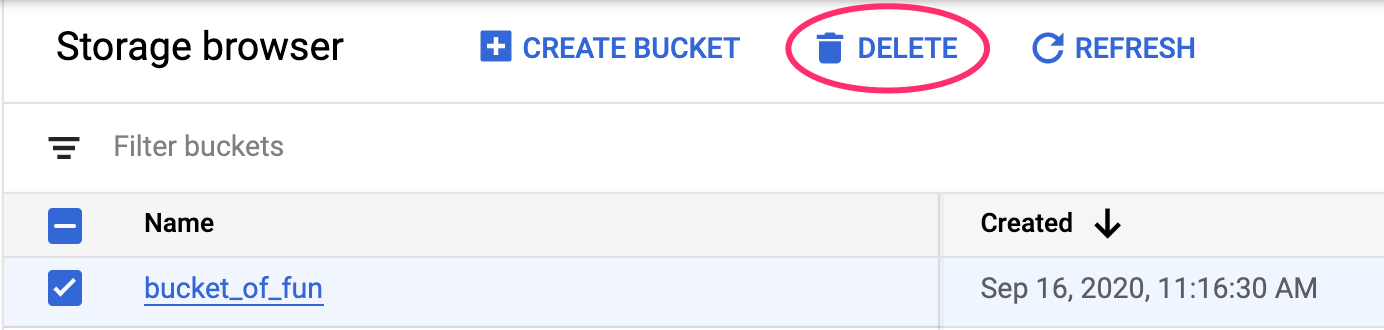# Vertex AI: Distributed hyperparameter tuning

## 1. Overview

In this lab, you'll learn how to use Vertex AI for hyperparameter tuning and distributed training. While this lab uses TensorFlow for the model code, the concepts are applicable to other ML frameworks as well.

## What you learn

You'll learn how to:

• Train a model using distributed training on a custom container
• Launch multiple trials of your training code for automated hyperparameter tuning

The total cost to run this lab on Google Cloud is about \$6 USD.

## 2. Intro to Vertex AI

This lab uses the newest AI product offering available on Google Cloud. Vertex AI integrates the ML offerings across Google Cloud into a seamless development experience. Previously, models trained with AutoML and custom models were accessible via separate services. The new offering combines both into a single API, along with other new products. You can also migrate existing projects to Vertex AI. If you have any feedback, please see the support page.

Vertex AI includes many different products to support end-to-end ML workflows. This lab will focus on Training and Workbench.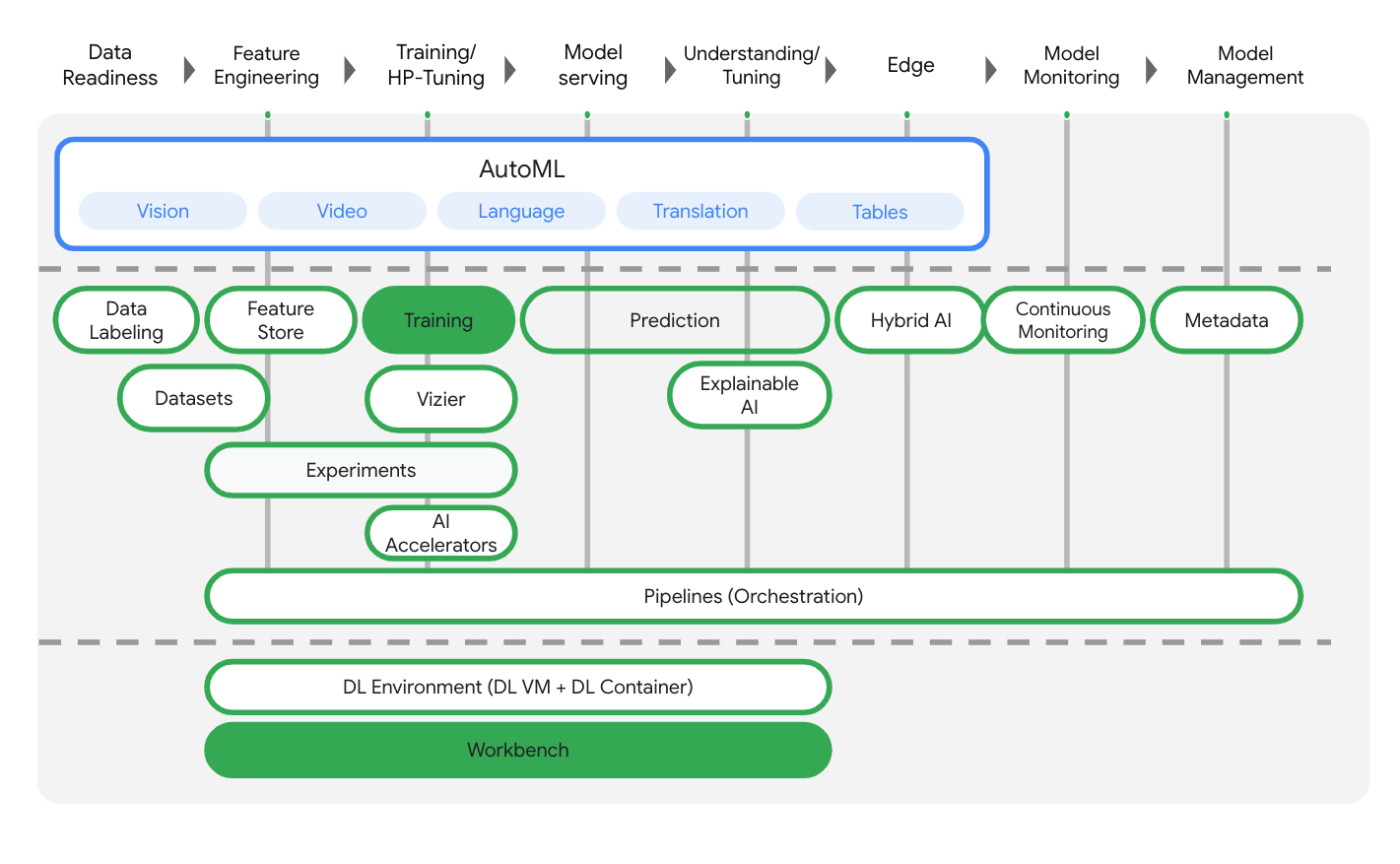## 3. Use Case Overview

In this lab, you'll use hyperparameter tuning to discover optimal parameters for an image classification model trained on the horses or humans dataset from TensorFlow Datasets.

#### Hyperparameter Tuning

Hyperparameter tuning with Vertex AI Training works by running multiple trials of your training application with values for your chosen hyperparameters, set within limits you specify. Vertex AI keeps track of the results of each trial and makes adjustments for subsequent trials.

To use hyperparameter tuning with Vertex AI Training, there are two changes you'll need to make to your training code:

1. Define a command-line argument in your main training module for each hyperparameter you want to tune.
2. Use the value passed in those arguments to set the corresponding hyperparameter in your application's code.

#### Distributed Training

If you have a single GPU, TensorFlow will use this accelerator to speed up model training with no extra work on your part. However, if you want to get an additional boost from using multiple GPUs, then you'll need to use `tf.distribute`, which is TensorFlow's module for running a computation across multiple devices.

This lab uses `tf.distribute.MirroredStrategy`, which you can add to your training applications with only a few code changes. This strategy creates a copy of the model on each GPU on your machine. The subsequent gradient updates will happen in a synchronous manner. This means that each GPU computes the forward and backward passes through the model on a different slice of the input data. The computed gradients from each of these slices are then aggregated across all of the GPUs and averaged in a process known as all-reduce. Model parameters are updated using these averaged gradients.

You don't need to know the details to complete this lab, but if you want to learn more about how distributed training works in TensorFlow, check out the video below:

## 4. Set up your environment

You'll need a Google Cloud Platform project with billing enabled to run this codelab. To create a project, follow the instructions here.

## Step 1: Enable the Compute Engine API

Navigate to Compute Engine and select Enable if it isn't already enabled.

## Step 2: Enable the Container Registry API

Navigate to the Container Registry and select Enable if it isn't already. You'll use this to create a container for your custom training job.

## Step 3: Enable the Vertex AI API

Navigate to the Vertex AI section of your Cloud Console and click Enable Vertex AI API.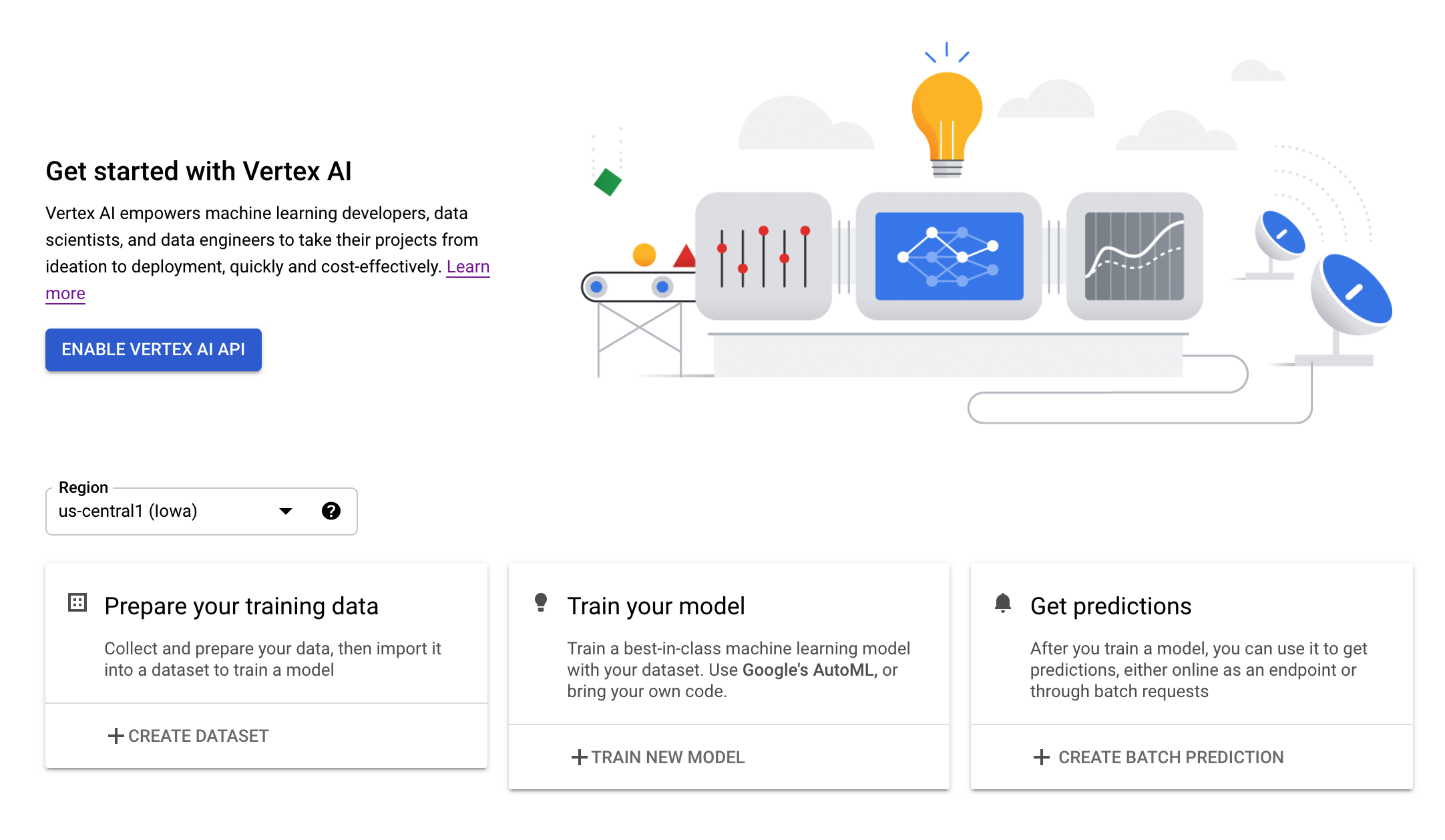## Step 4: Create a Vertex AI Workbench instance

From the Vertex AI section of your Cloud Console, click on Workbench: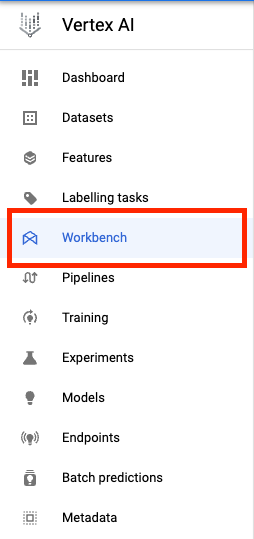Enable the Notebooks API if it isn't already.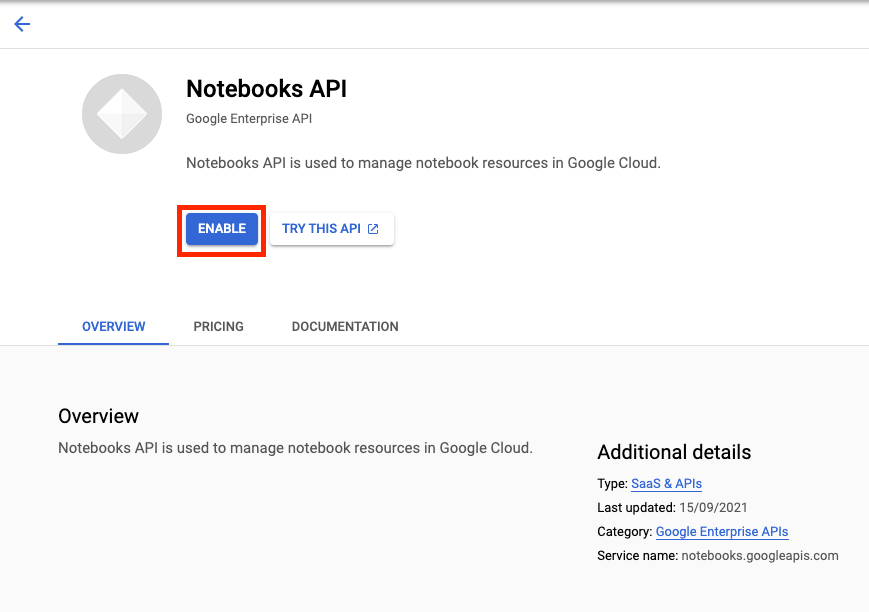Once enabled, click MANAGED NOTEBOOKS: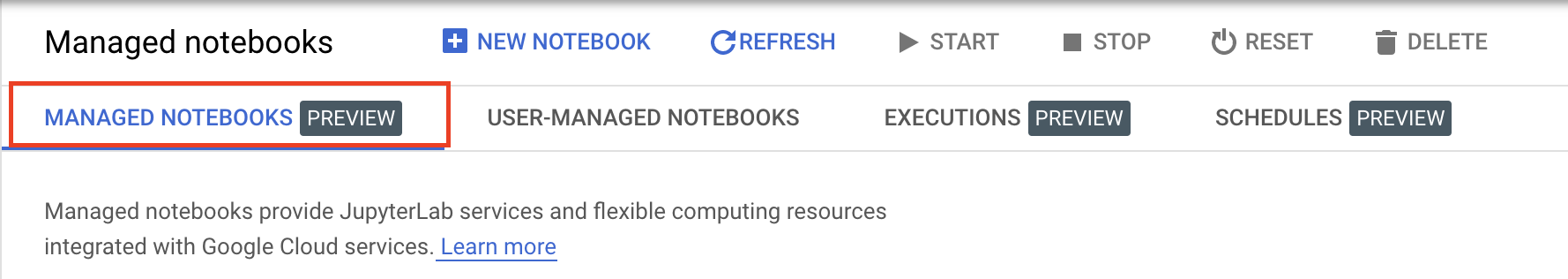Then select NEW NOTEBOOK.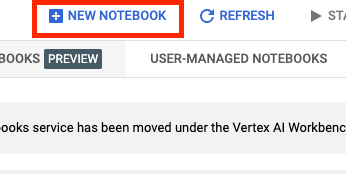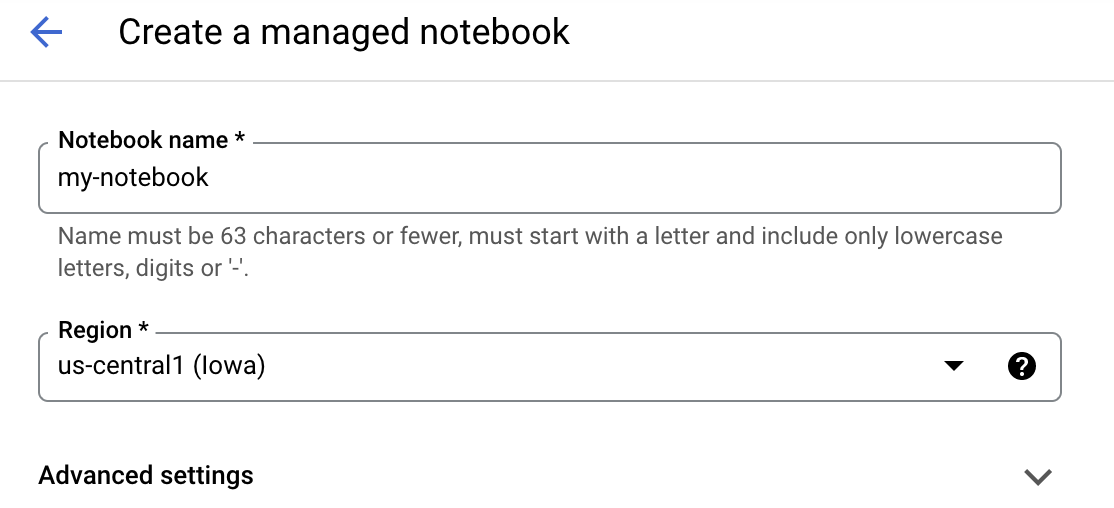Under Advanced Settings, enable idle shutdown and set the number of minutes to 60. This means your notebook will shutdown automatically when not in use so you don't incur unnecessary costs.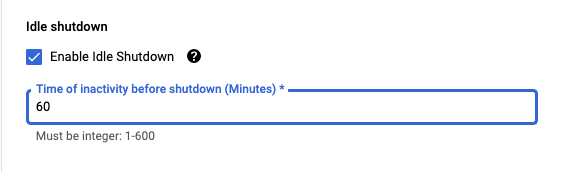Under Security select "Enable terminal" if it is not already enabled.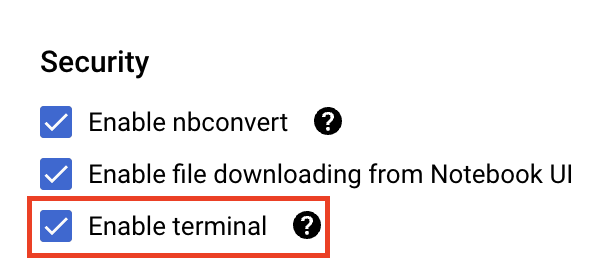You can leave all of the other advanced settings as is.

Next, click Create. The instance will take a couple minutes to be provisioned.

Once the instance has been created, select Open JupyterLab.The first time you use a new instance, you'll be asked to authenticate. Follow the steps in the UI to do so.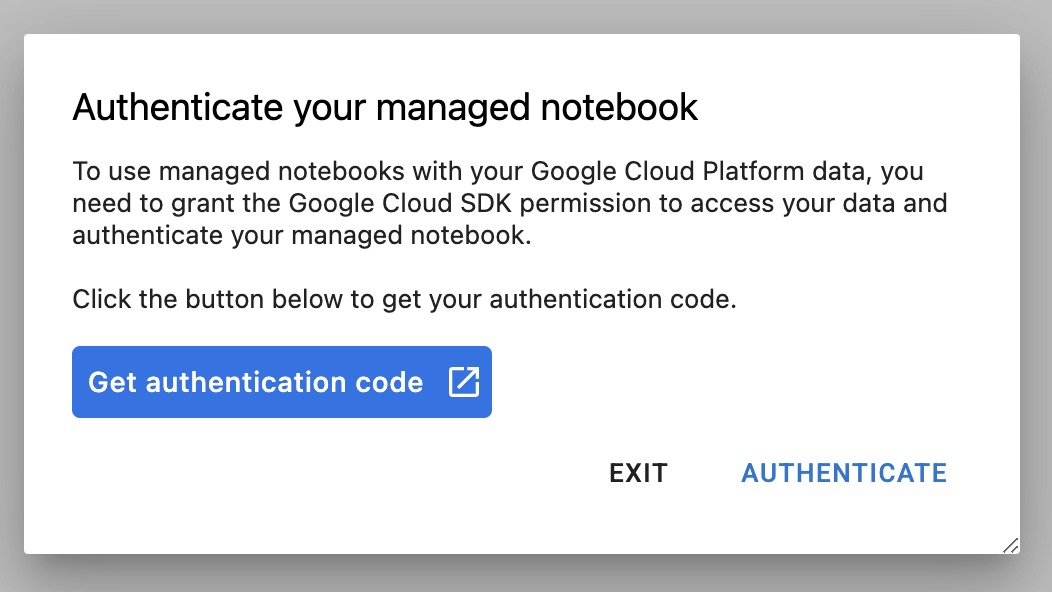## 5. Write training code

To start, from the Launcher menu, open a Terminal window in your notebook instance: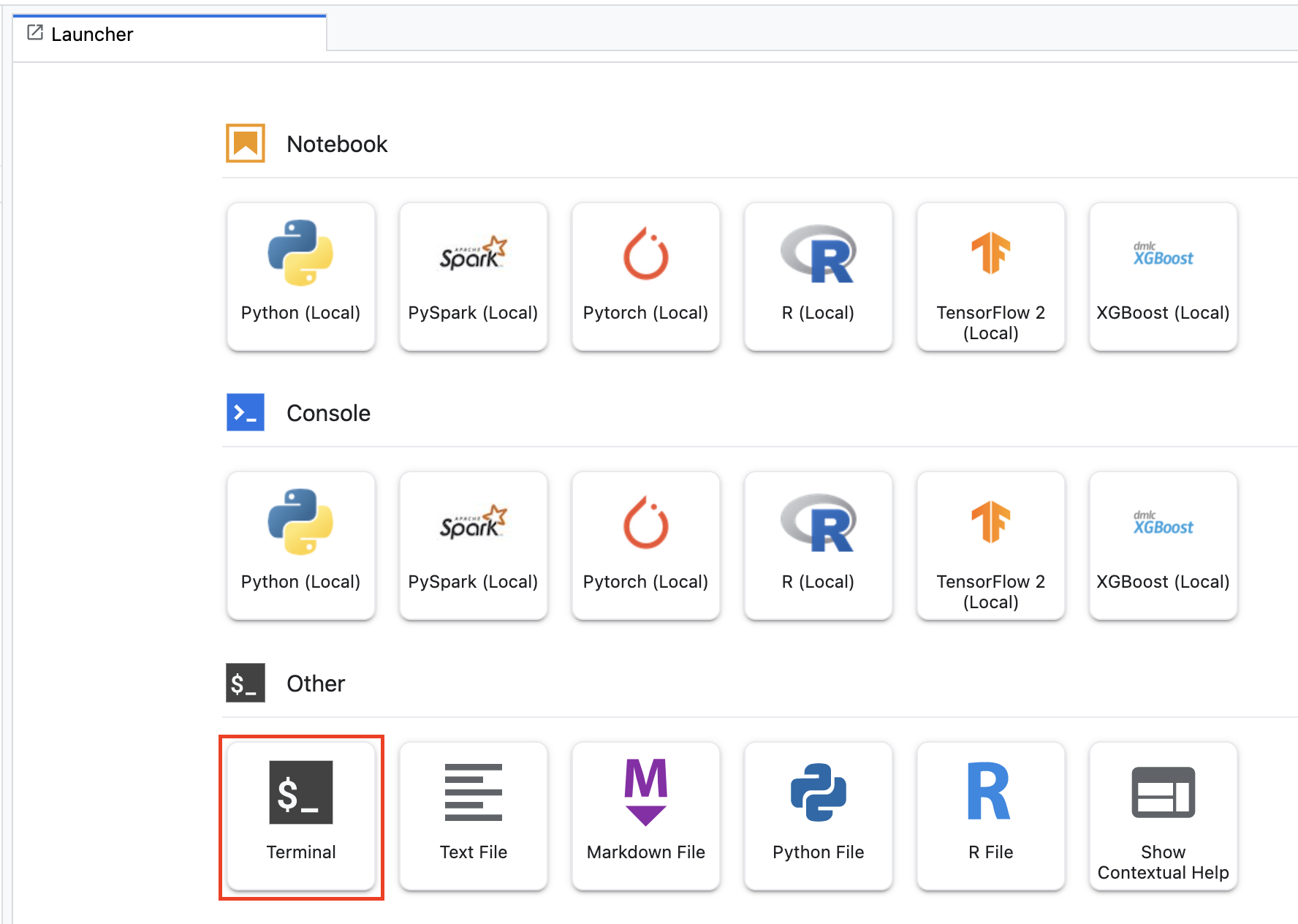Create a new directory called `vertex-codelab` and cd into it.

``````mkdir vertex-codelab
cd vertex-codelab
``````

Run the following to create a directory for the training code and a Python file where you'll add the code:

``````mkdir trainer
``````

You should now have the following in your `vertex-codelab` directory:

``````+ trainer/
``````

Next, open the `task.py` file you just created and paste in all of the code below.

``````import tensorflow as tf
import tensorflow_datasets as tfds
import argparse
import hypertune
import os

NUM_EPOCHS = 10
BATCH_SIZE = 64

def get_args():
'''Parses args. Must include all hyperparameters you want to tune.'''

parser = argparse.ArgumentParser()
'--learning_rate',
required=True,
type=float,
help='learning rate')
'--momentum',
required=True,
type=float,
help='SGD momentum value')
'--num_units',
required=True,
type=int,
help='number of units in last hidden layer')
args = parser.parse_args()
return args

def preprocess_data(image, label):
'''Resizes and scales images.'''

image = tf.image.resize(image, (150,150))
return tf.cast(image, tf.float32) / 255., label

def create_dataset(batch_size):
'''Loads Horses Or Humans dataset and preprocesses data.'''

data, info = tfds.load(name='horses_or_humans', as_supervised=True, with_info=True)

# Create train dataset
train_data = data['train'].map(preprocess_data)
train_data  = train_data.shuffle(1000)
train_data  = train_data.batch(batch_size)

# Create validation dataset
validation_data = data['test'].map(preprocess_data)
validation_data  = validation_data.batch(batch_size)

return train_data, validation_data

def create_model(num_units, learning_rate, momentum):
'''Defines and compiles model.'''

inputs = tf.keras.Input(shape=(150, 150, 3))
x = tf.keras.layers.Conv2D(16, (3, 3), activation='relu')(inputs)
x = tf.keras.layers.MaxPooling2D((2, 2))(x)
x = tf.keras.layers.Conv2D(32, (3, 3), activation='relu')(x)
x = tf.keras.layers.MaxPooling2D((2, 2))(x)
x = tf.keras.layers.Conv2D(64, (3, 3), activation='relu')(x)
x = tf.keras.layers.MaxPooling2D((2, 2))(x)
x = tf.keras.layers.Flatten()(x)
x = tf.keras.layers.Dense(num_units, activation='relu')(x)
outputs = tf.keras.layers.Dense(1, activation='sigmoid')(x)
model = tf.keras.Model(inputs, outputs)
model.compile(
loss='binary_crossentropy',
optimizer=tf.keras.optimizers.SGD(learning_rate=learning_rate, momentum=momentum),
metrics=['accuracy'])
return model

def main():
args = get_args()

# Create distribution strategy
strategy = tf.distribute.MirroredStrategy()

# Get data
GLOBAL_BATCH_SIZE = BATCH_SIZE * strategy.num_replicas_in_sync
train_data, validation_data = create_dataset(GLOBAL_BATCH_SIZE)

# Wrap variable creation within strategy scope
with strategy.scope():
model = create_model(args.num_units, args.learning_rate, args.momentum)

# Train model
history = model.fit(train_data, epochs=NUM_EPOCHS, validation_data=validation_data)

# Define metric
hp_metric = history.history['val_accuracy'][-1]

hpt = hypertune.HyperTune()
hpt.report_hyperparameter_tuning_metric(
hyperparameter_metric_tag='accuracy',
metric_value=hp_metric,
global_step=NUM_EPOCHS)

if __name__ == "__main__":
main()
``````

Let's take a deeper look at the code and examine the components specific to distributed training and hyperparameter tuning.

### Distributed Training

1. In the `main()` function, the `MirroredStrategy` object is created. Next, you wrap the creation of your model variables within the strategy's scope. This step tells TensorFlow which variables should be mirrored across the GPUs.
2. The batch size is scaled up by the `num_replicas_in_sync`. Scaling the batch size is a best practice when using synchronous data parallelism strategies in TensorFlow. You can learn more here.

### Hyperparameter Tuning

1. The script imports the `hypertune` library. Later when we build the container image, we'll need to make sure we install this library.
2. The function `get_args()` defines a command-line argument for each hyperparameter you want to tune. In this example, the hyperparameters that will be tuned are the learning rate, the momentum value in the optimizer, and the number of units in the last hidden layer of the model, but feel free to experiment with others. The value passed in those arguments is then used to set the corresponding hyperparameter in the code (eg, set `learning_rate = args.learning_rate`)
3. At the end of the `main()` function, the `hypertune` library is used to define the metric you want to optimize. In TensorFlow, the Keras `model.fit` method returns a `History` object. The `History.history` attribute is a record of training loss values and metrics values at successive epochs. If you pass validation data to `model.fit` the `History.history` attribute will include validation loss and metrics values as well. For example, if you trained a model for three epochs with validation data and provided `accuracy` as a metric, the `History.history` attribute would look similar to the following dictionary.
``````{
"accuracy": [
0.7795261740684509,
0.9471358060836792,
0.9870933294296265
],
"loss": [
0.6340447664260864,
0.16712145507335663,
0.04546636343002319
],
"val_accuracy": [
0.3795261740684509,
0.4471358060836792,
0.4870933294296265
],
"val_loss": [
2.044623374938965,
4.100203514099121,
3.0728273391723633
]
``````

If you want the hyperparameter tuning service to discover the values that maximize the model's validation accuracy, you define the metric as the last entry (or `NUM_EPOCS - 1`) of the `val_accuracy` list. Then, pass this metric to an instance of `HyperTune`. You can pick whatever string you like for the `hyperparameter_metric_tag`, but you'll need to use the string again later when you kick off the hyperparameter tuning job.

## 6. Containerize code

The first step in containerizing your code is to create a Dockerfile. In the Dockerfile you'll include all the commands needed to run the image. It'll install all the necessary libraries and set up the entry point for the training code.

## Step 1: Write Dockerfile

From your Terminal, make sure you're in the `vertex-codelab` directory and create an empty Dockerfile:

``````touch Dockerfile
``````

You should now have the following in your `vertex-codelab` directory:

``````+ Dockerfile
+ trainer/
``````

Open the Dockerfile and copy the following into it:

``````FROM gcr.io/deeplearning-platform-release/tf2-gpu.2-7

WORKDIR /

# Installs hypertune library
RUN pip install cloudml-hypertune

# Copies the trainer code to the docker image.
COPY trainer /trainer

# Sets up the entry point to invoke the trainer.
``````

This Dockerfile uses the Deep Learning Container TensorFlow Enterprise 2.7 GPU Docker image. The Deep Learning Containers on Google Cloud come with many common ML and data science frameworks pre-installed. After downloading that image, this Dockerfile sets up the entrypoint for the training code.

## Step 2: Build the container

From your Terminal, run the following to define an env variable for your project, making sure to replace `your-cloud-project` with the ID of your project:

``````PROJECT_ID='your-cloud-project'
``````

Define a variable with the URI of your container image in Google Container Registry:

``````IMAGE_URI="gcr.io/\$PROJECT_ID/horse-human-codelab:latest"
``````

Configure Docker

``````gcloud auth configure-docker
``````

Then, build the container by running the following from the root of your `vertex-codelab` directory:

``````docker build ./ -t \$IMAGE_URI
``````

Lastly, push it to Google Container Registry:

``````docker push \$IMAGE_URI
``````

## Step 3: Create a Cloud Storage bucket

In our training job, we'll pass in the path to a staging bucket.

Run the following in your Terminal to create a new bucket in your project.

``````BUCKET_NAME="gs://\${PROJECT_ID}-hptune-bucket"
gsutil mb -l us-central1 \$BUCKET_NAME
``````

## Step 1: Create custom training job with hyperparameter tuning

From the launcher, open up a new TensorFlow 2 Notebook.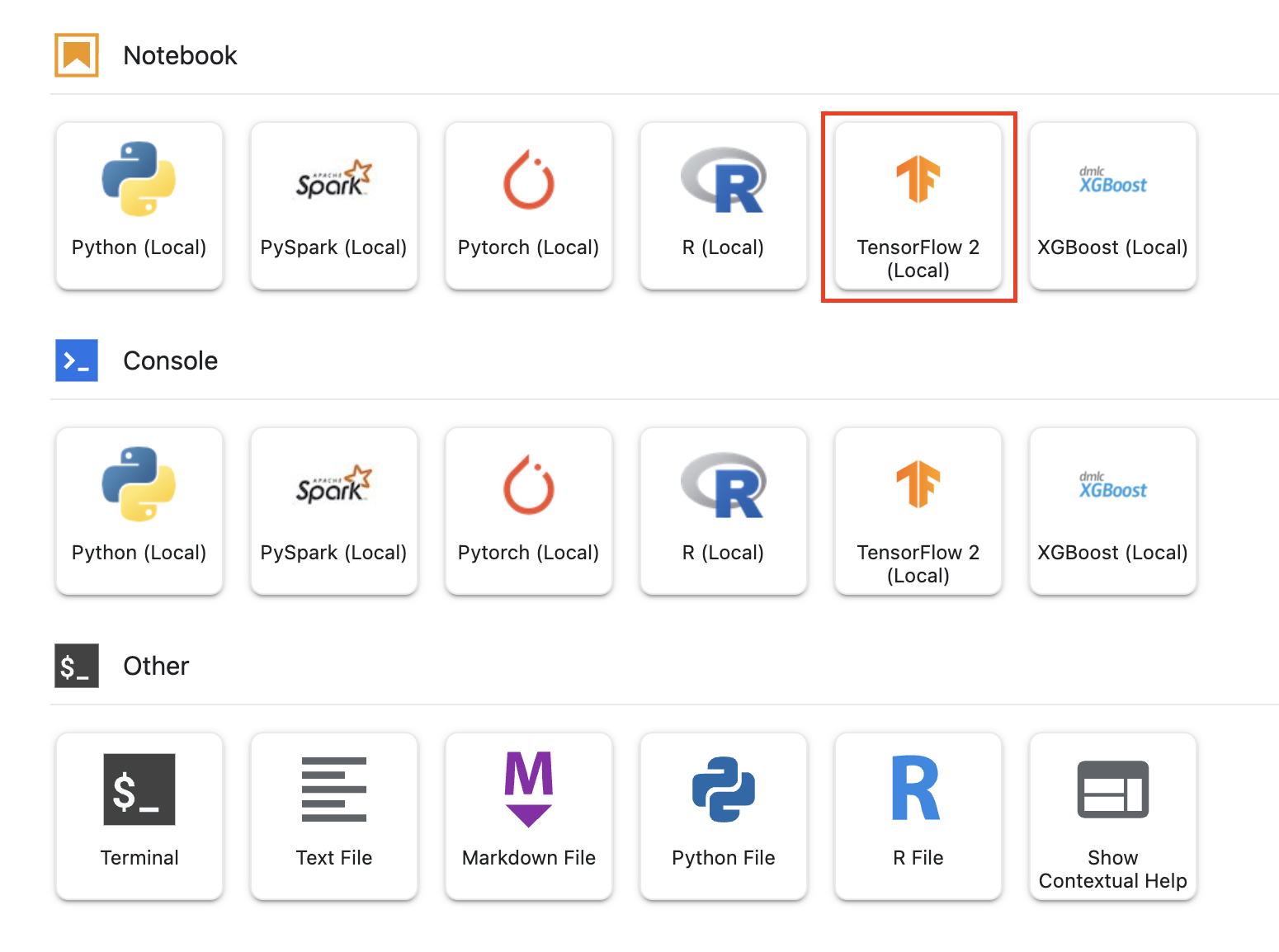Import the Vertex AI Python SDK.

``````from google.cloud import aiplatform
from google.cloud.aiplatform import hyperparameter_tuning as hpt
``````

To launch the hyperparameter tuning job, you need to first define the `worker_pool_specs`, which specifies the machine type and Docker image. The following spec defines one machine with two NVIDIA Tesla V100 GPUs.

You'll need to replace `{PROJECT_ID}` in the `image_uri` with your project.

``````# The spec of the worker pools including machine type and Docker image
# Be sure to replace PROJECT_ID in the "image_uri" with your project.

worker_pool_specs = [{
"machine_spec": {
"machine_type": "n1-standard-4",
"accelerator_type": "NVIDIA_TESLA_V100",
"accelerator_count": 2
},
"replica_count": 1,
"container_spec": {
"image_uri": "gcr.io/{PROJECT_ID}/horse-human-codelab:latest"
}
}]

``````

Next, define the `parameter_spec`, which is a dictionary specifying the parameters you want to optimize. The dictionary key is the string you assigned to the command line argument for each hyperparameter, and the dictionary value is the parameter specification.

For each hyperparameter, you need to define the Type as well as the bounds for the values that the tuning service will try. Hyperparameters can be of type Double, Integer, Categorical, or Discrete. If you select the type Double or Integer, you'll need to provide a minimum and maximum value. And if you select Categorical or Discrete you'll need to provide the values. For the Double and Integer types, you'll also need to provide the Scaling value. You can learn more about how to pick the best scale in this video.

``````# Dictionary representing parameters to optimize.
# The dictionary key is the parameter_id, which is passed into your training
# job as a command line argument,
# And the dictionary value is the parameter specification of the metric.
parameter_spec = {
"learning_rate": hpt.DoubleParameterSpec(min=0.001, max=1, scale="log"),
"momentum": hpt.DoubleParameterSpec(min=0, max=1, scale="linear"),
"num_units": hpt.DiscreteParameterSpec(values=[64, 128, 512], scale=None)
}
``````

The final spec to define is `metric_spec`, which is a dictionary representing the metric to optimize. The dictionary key is the `hyperparameter_metric_tag` that you set in your training application code, and the value is the optimization goal.

``````# Dicionary representing metrics to optimize.
# The dictionary key is the metric_id, which is reported by your training job,
# And the dictionary value is the optimization goal of the metric.
metric_spec={'accuracy':'maximize'}
``````

Once the specs are defined, you'll create a `CustomJob`, which is the common spec that will be used to run your job on each of the hyperparameter tuning trials.

You'll need to replace `{YOUR_BUCKET}` with the bucket you created earlier.

``````# Replace YOUR_BUCKET
my_custom_job = aiplatform.CustomJob(display_name='horses-humans',
worker_pool_specs=worker_pool_specs,
staging_bucket='gs://{YOUR_BUCKET}')
``````

Then, create and run the `HyperparameterTuningJob`.

``````hp_job = aiplatform.HyperparameterTuningJob(
display_name='horses-humans',
custom_job=my_custom_job,
metric_spec=metric_spec,
parameter_spec=parameter_spec,
max_trial_count=6,
parallel_trial_count=2,
search_algorithm=None)

hp_job.run()
``````

There are a few arguments to note:

• max_trial_count: You'll need to put an upper bound on the number of trials the service will run. More trials generally leads to better results, but there will be a point of diminishing returns, after which additional trials have little or no effect on the metric you're trying to optimize. It is a best practice to start with a smaller number of trials and get a sense of how impactful your chosen hyperparameters are before scaling up.
• parallel_trial_count: If you use parallel trials, the service provisions multiple training processing clusters. Increasing the number of parallel trials reduces the amount of time the hyperparameter tuning job takes to run; however, it can reduce the effectiveness of the job overall. This is because the default tuning strategy uses results of previous trials to inform the assignment of values in subsequent trials.
• search_algorithm: You can set the search algorithm to grid, random, or default (None). The default option applies Bayesian optimization to search the space of possible hyperparameter values and is the recommended algorithm. You can learn more about this algorithm here.

Once the job kicks off, you'll be able to track the status in the UI under the HYPERPARAMETER TUNING JOBS tab.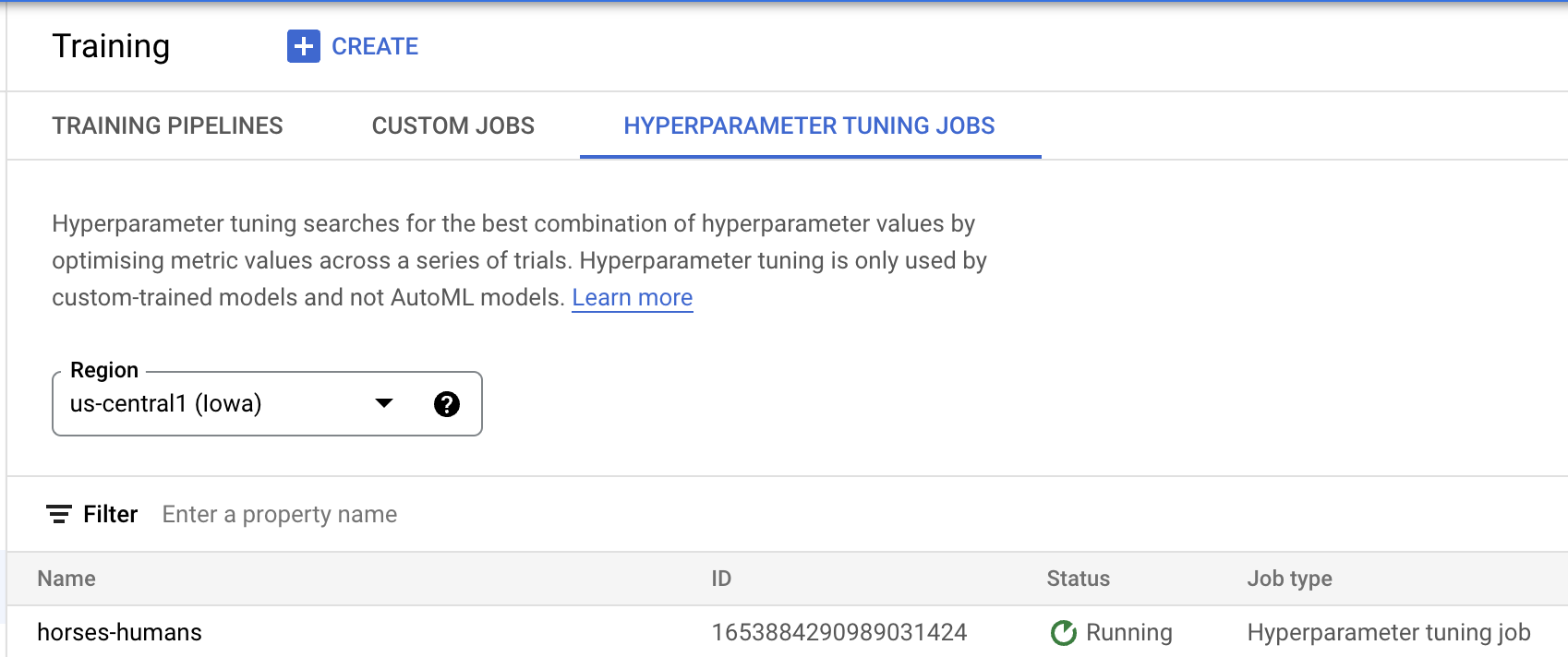Once the job completes, you can view and sort the results of your trials to discover the best combination of hyperparameter values.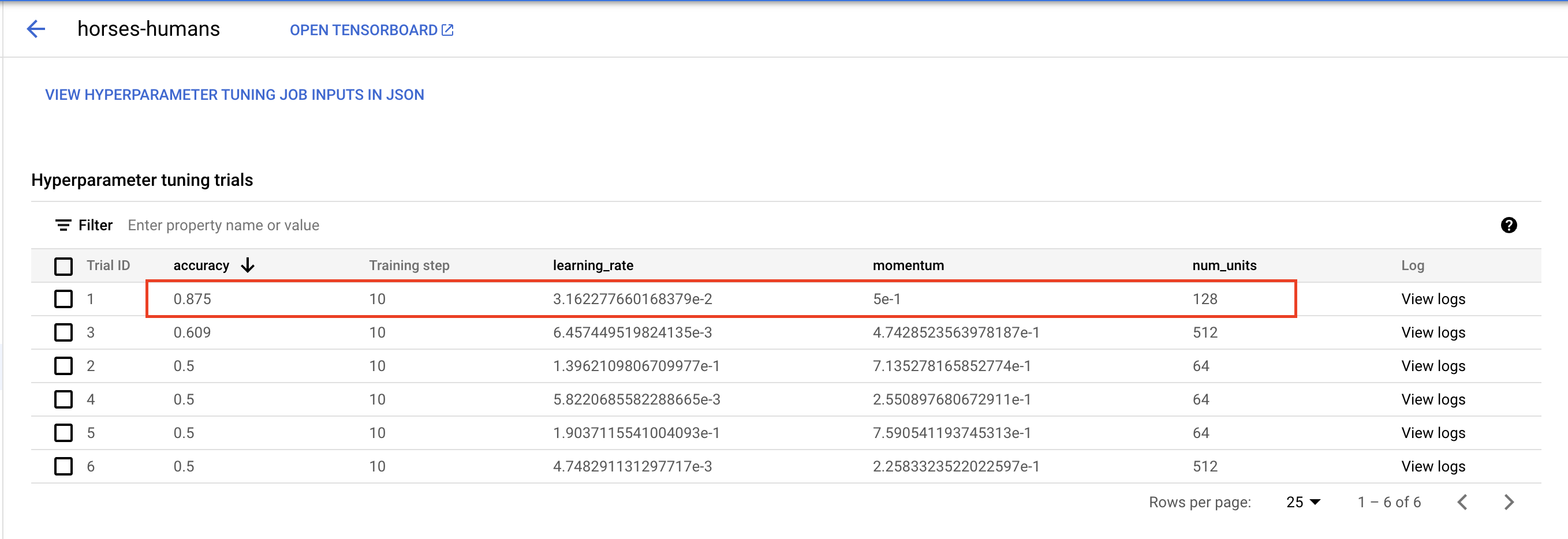🎉 Congratulations! 🎉

You've learned how to use Vertex AI to:

• Run a hyperparameter tuning job with distributed training